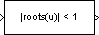# Polynomial Stability Test

Use Schur-Cohn algorithm to determine whether all roots of input polynomial are inside unit circle

## Library

Math Functions / Polynomial Functions

`dsppolyfun`

•## Description

The Polynomial Stability Test block uses the Schur-Cohn algorithm to determine whether all roots of a polynomial are within the unit circle.

```y = all(abs(roots(u)) < 1) % Equivalent MATLAB code ```

Each column of the M-by-N input matrix u contains M coefficients from a distinct polynomial,

`$f\left(x\right)={u}_{1}{x}^{M-1}+{u}_{2}{x}^{M-2}+\dots +{u}_{M}$`

arranged in order of descending exponents, u1, u2, ..., uM. The polynomial has order M-1 and positive integer exponents.

Inputs to the block represent the polynomial coefficients as shown in the previous equation. The block always treats length-M unoriented vector input as an M-by-1 matrix.

The output is a 1-by-N matrix with each column containing the value `1` or `0`. The value `1` indicates that the polynomial in the corresponding column of the input is stable; that is, the magnitudes of all solutions to f(x) = 0 are less than 1. The value `0` indicates that the polynomial in the corresponding column of the input might be unstable; that is, the magnitude of at least one solution to f(x) = 0 is greater than or equal to 1.

### Applications

This block is most commonly used to check the pole locations of the denominator polynomial, A(z), of a transfer function, H(z).

`$H\left(z\right)=\frac{B\left(z\right)}{A\left(z\right)}=\frac{{b}_{1}+{b}_{2}{z}^{-1}+\dots +{b}_{m}{z}^{-\left(m-1\right)}}{{a}_{1}+{a}_{2}{z}^{-1}+\dots +{a}_{n}{z}^{-\left(n-1\right)}}$`

The poles are the n-1 roots of the denominator polynomial, A(z). When any poles are located outside the unit circle, the transfer function H(z) is unstable. As is typical in DSP applications, the transfer function above is specified in descending powers of z-1 rather than z.

## Supported Data Types

• Double-precision floating point

• Single-precision floating point

• Boolean — Block outputs are always Boolean.

 Least Squares Polynomial Fit DSP System Toolbox Polynomial Evaluation DSP System Toolbox `polyfit` MATLAB